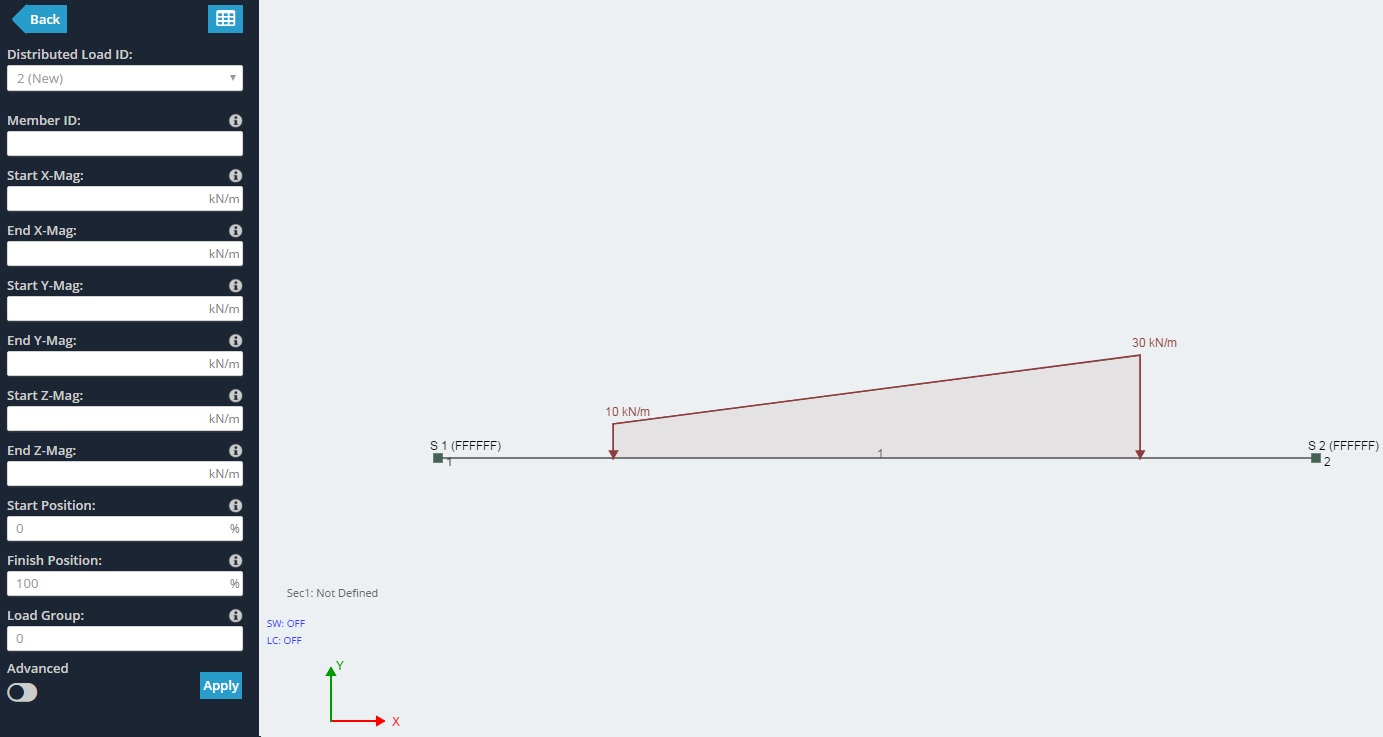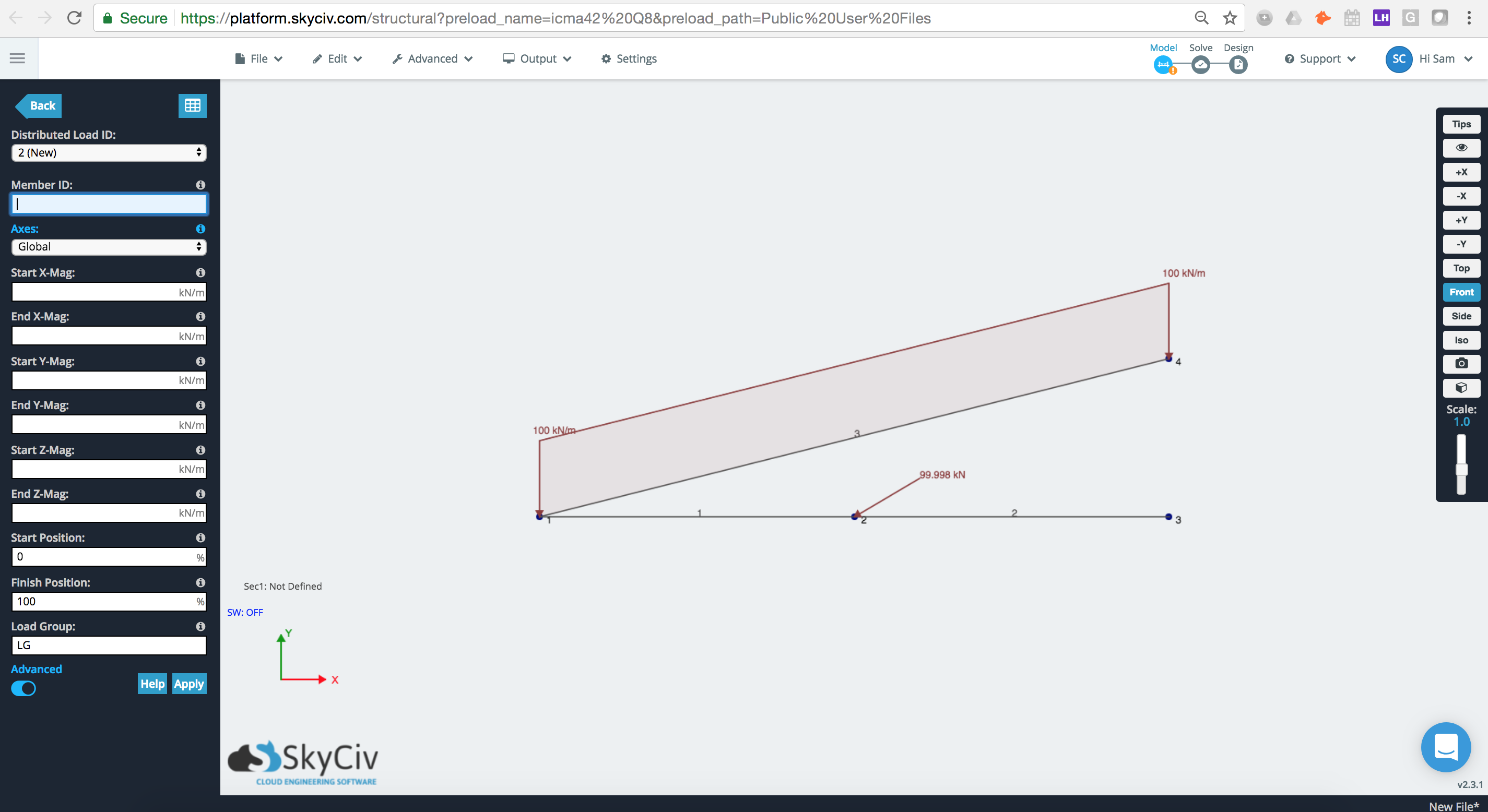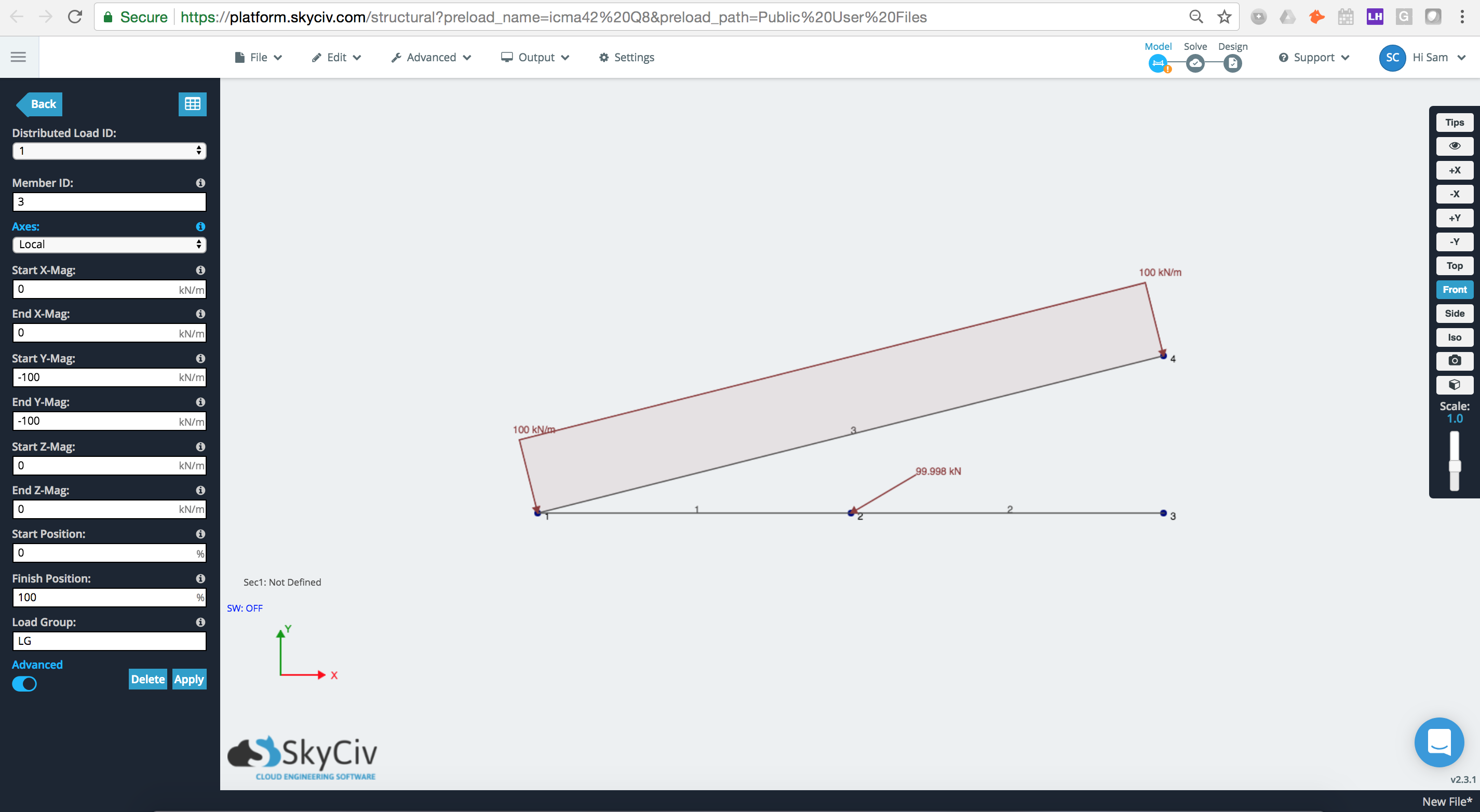Documentation [Deprecated]
SkyCiv Documentation

Getting Started

Section Builder
Operations
Built-Up and Composite Sections
Custom Shapes

Member Design
General
Member Design Modules
Code Verification

RC Design
General
Code Verification

Distributed loads are forces that act over a span and are measured in force per unit of length (e.g. kN/m). They can be either uniform or non-uniform.

DL's are applied to a member and by default will span the entire length of the member. Users however have the option to specify the start and end of the DL somewhere along the span.

DL's which are applied at an angle to the member can be specified by providing the X,Y,Z components.

To apply a DL, simply specify values for:

• Distributed Load ID - The numerical ID used to identify each DL.
• Member ID - The member where the DL is applied.
• Start X-Mag - The starting magnitude of the DL - this can be either in the global X direction, or the member's local X direction by changing the axis reference point.
• End X-Mag - The ending magnitude of the DL - this can be either in the global X direction, or the member's local X direction by changing the axis reference point.
• Start Y-Mag - The starting magnitude of the DL - this can be either in the global Y direction, or the member's local Y direction by changing the axis reference point.
• End Y-Mag - The ending magnitude of the DL - this can be either in the global Y direction, or the member's local Y direction by changing the axis reference point.
• Start Z-Mag - The starting magnitude of the DL in the Z direction - again this can be either Local Z or the member's Local Z, depending on the Axis setting.
• End Z-Mag - The ending magnitude of the DL in the Z direction - again this can be either Local Z or the member's Local Z, depending on the Axis setting.
• Start Position - The position along the member where the DL starts. Expressed as a %.
• Finish Position - The position along the member where the DL ends. Expressed as a %.

• Axes - Apply the DL along the global (default) or local axes. If Local, it may help to toggle on the Show Member Local Axis ON in your Visibility Settings, so that users can see the reference axis.

## Example:

Here's an example where the distributed load has a -10kN/m Start Y magnitude and a -30kN/m end Y magnitude. It also has a 20% start position and an 80% end position - showing that it does not extend the entire span of the member, but rather it starts 20% from the start and end node (1 and 2 respectively).## Local/Global Axes

### Global Axis

Here is an example of where member 3 has a 100kN/m distibuted load applied to its Global axis. We can see the force here is applied directly in the global Y (down).### Local Axis

If we change the axes option to Local we can see that the distributed load has now been applied to the member's local axis, where local Y is directly perpendicular to the member.